# Zinc Cyanide Plating Process

In order to secure definite information regarding the best conditions of operation of plating solutions, it is necessary first to obtain reliable methods for preparing and analyzing solutions of any given composition. This need has been especially felt in connection with such mixtures as the zinc cyanide plating solutions in which, as will be explained shortly, a given composition can be produced by different methods, and for which the methods of analysis in ordinary use may be wholly inadequate.

When zinc cyanide Zn (CN)2 is dissolved in sodium cyanide NaCN, a double compound, sodium zinc cyanide Zn(CN)2. 2NaCN is formed by simple addition, which may be represented thus:

Zn(CN)2 + 2NaCN = Zn(CN) 2·2NaCN or Na2Zn(CN) 4

Similarly, when zinc hydroxide, Zn(OH)2 (or zinc oxide, ZnO) is dissolved in sodium hydroxide (caustic soda, NaOH) a double compound, sodium zincate, Na2ZnO2 or Zn(ONa)2 is formed,

Zn(OH)2 + 2NaOH = Zn(OH)2 . 2NaOH = Zn(ONa)2 + 2H2O or
ZnO + 2NaOH = Zn(ONa)2 +H2O

It is also possible to dissolve zinc cyanide in sodium hydroxide, or to dissolve zinc oxide in sodium cyanide. In each case the same product is formed; that is, a mixture of sodium zinc cyanide and sodium zincate.

2Zn(CN) 2 + 4NaOH = Zn(CN)2 . 2NaCN + Zn(ONa)2 + 2H2O
2H2O + 2ZnO + 4NaCN = 2Zn(CN) 2 + 4NaOH = Zn(CN)2 . 2NaCN + Zn(ONa) 2 + 2H2O

From these considerations, it is obvious that when, as is usually the case, it is desired to have considerable free alkali in a zinc cyanide solution, a solution identical in all respects can be prepared by the substitution of zinc oxide for all or a part of the zinc cyanide, and the use of a proportionately greater amount of sodium cyanide, and less amount of sodium hydroxide. The net saving of such a substitution is represented by the economy of adding zinc in, the form of a zinc oxide instead of zinc cyanide, and of adding cyanide in the form of sodium cyanide instead of zinc cyanide. The question of whether satisfactory solutions may be prepared with less total cyanide was not considered in these experiments and is not necessarily connected with such a substitution.

## PREPARATION OF SOLUTIONS

The following approximate factors showing the relations by weight between the various constituents are used in the subsequent calculations:

Zinc cyanide x o.56=zinc.
Zinc cyanide x 0.69=zinc oxide.
Zinc cyanide x .o.68=equivalent sodium hydroxide.
Zinc cyanide x o.84=equivalent sodium cyanide.
Zinc x 1.8o=zinc cyanide.
Zinc x 1.24=zinc oxide.
Zinc oxide x 0.80=zinc.
Sodium hydroxide x 1.23=equivalent sodium cyanide.
Sodium cyanide x o.83 =equivalent sodium hydroxide.
Grams per liter x o.134=ounces per gallon.

When zinc oxide is dissolved in sodium cyanide an equivalent amount of sodium hydroxide, almost exactly equal to the weight of the zinc oxide used, is liberated. In order therefore to determine how much zinc cyanide in a plating solution may be substituted by zinc oxide, all that is necessary is to multiply the desired zinc cyanide content by 0.69 or for practical purposes 0.7, giving the equivalent amount of zinc oxide. If this amount is equal to or less than the desired amount of sodium hydroxide, the entire amount of zinc oxide indicated may be used. Should this amount be greater than the desired amount of sodium hydroxide, only as much zinc oxide may be used as the desired sodium hydroxide content. In the latter case sufficient zinc cyanide must also be added to furnish the desired zinc content.

The additional amount of sodium cyanide to be used is easily determined by multiplying the amount of zinc cyanide to be replaced by zinc oxide, by 0.84. The total cyanide to be used will then be equal to this plus the original content of sodium cyanide.

The amount of sodium hydroxide required is simply the originally specified amount minus the amount of zinc oxide used.

Examples:
Suppose it is desired to prepare the following two solutions by the use of zinc oxide: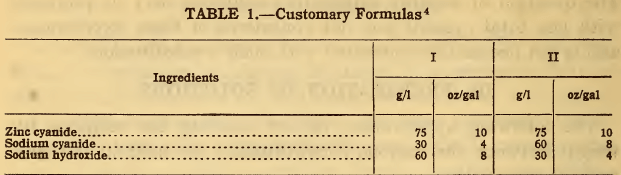The amount of zinc oxide equivalent to the zinc cyanide is equal to 75 g/l x 0.7 = 52.5 g/l. The use of this amount of zinc oxide would liberate an equal amount of sodium hydroxide. In solution I, where 60 g/l of sodium hydroxide is desired, the full amount of sodium hydroxide will be required. In solution II where it is desired to have 30 g/l of sodium hydroxide, only that amount—that is, 30 g/l of zinc oxide—may be used instead of 52.5 g/l.

Consequently only 30/52.5  x 75 = 43 g/l of the zinc cyanide can be substituted, leaving 32 g/l of zinc cyanide to be added. The amount of sodium cyanide to be used in addition to that originally specified is 0.84 times the amount of zinc cyanide replaced; that is, 0.84 x 75=63 g/l in solution I and 0.84 x 43 = 36 g/l in solution II. The revised formulas will then be as follows:

In the preparation of such solutions it is necessary to first dissolve the sodium cyanide and hydroxide in one-half or less of the final volume of water and then to slowly pour the zinc oxide, previously mixed with water to form a thin paste, into the first solution while stirring. When the solution is complete, additional water may be added to produce the desired volume or specific gravity.

The exact economy to be effected by such substitutions will depend upon the market prices of the chemicals involved, and can be readily computed for such examples as are given. In this connection it should be borne in mind that zinc oxide contains 80 per cent of metallic zinc as against 56 per cent in zinc cyanide; and that sodium cyanide contains 53 per cent of cyanogen (CN) as against 45 per cent in zinc cyanide. Solutions prepared by this method have been found to produce results equal to those obtained in solutions of similar composition prepared from zinc cyanide.

## ANALYSIS OF CYANIDE SOLUTIONS

### PRINCIPLES

While the methods for the determination of zinc in plating solutions are comparatively simple and reliable, the methods frequently employed for the determination of free cyanide and free alkali yield such erratic results that they are of little or no value. The principal cause of uncertainty in such methods is that they attempt to determine two quantities which vary with dilution and which indeed can scarcely be said to exist. By the methods to be described in this paper it is possible to determine the three principal constituents, viz, total zinc, total cyanide, and total alkali. From these values may be calculated, if desired, the excess of one or another constituent, although for control purposes such calculations may not be necessary.

As explained above, the principal compounds existing in zinc cyanide plating solutions are sodium zinc cyanide Zn(CN)2. 2NaCN and sodium zincate Zn(ONa)2, together with any excess of sodium cyanide or sodium hydroxide that may be present. If such compounds were stable—that is, difficulty decomposable— the analysis of such solutions would be comparatively simple. As a matter of fact, however, they are readily dissociated or decomposed by the action of water itself so that even dilution will change their relative amounts.

This decomposition may take place from either one or both of two causes, viz, dissociation and hydrolysis, both of which are reversible; that is, the reactions may take place in either direction. The extent to which such changes take place is determined by the various conditions such as concentration (or dilution), temperature, etc.

“Dissociation” is simply the breaking up of a compound into elements or simpler compounds. For example, if it were possible to prepare in a dry state sodium zinc cyanide, having the formula Zn(CN)2.2NaCN, this compound when dissolved in water would decompose in part into zinc cyanide and sodium cyanide, the decomposition taking place to a greater extent the greater the proportion of water used. If more sodium cyanide were then added to the solution, the reaction would be reversed and more of the double compound would be formed. Conversely, if some of the sodium cyanide first liberated is removed from the solution, the decomposition continues and more sodium cyanide is set free. The above facts are all expressed in the reversible chemical equation.

Zn(CN) 2.2NaCN↔Zn(CN)2 + 2NaCN

in which the double arrow shows that, depending upon the conditions, the reaction may take place in either direction.

“Hydrolysis” is the decomposition of a compound brought about by the presence of water, which actually enters into the chemical reaction which takes place. As is well known, when an acid and a base react with each other a salt and water are formed. Salts formed from a weak acid and a strong base, or vice versa, when dissolved in water are decomposed to some extent by the water into the original acid and the base. These are liberated in equivalent quantities, but if the base is stronger than the acid, the solution is alkaline toward the customary indicators such as litmus, phenolphthalein, etc.; while if the acid is stronger, the solution is acid to indicators. Thus when sodium cyanide is dissolved in water the solution is alkaline, because (chemically) hydrocyanic acid, HCN, is a weak acid, while sodium hydroxide is a strong base. The reaction may be expressed thus:

NaCN + H2O↔NaOH + HCN

Similarly sodium zincate when dissolved in water is alkaline, because zinc hydroxide behaves as a weak acid in such salts as sodium zincate.

If, therefore, an attempt is made to determine the excess of sodium cyanide in a zinc solution—for example, by titration with silver nitrate—more cyanide is liberated as the free cyanide is used up by the silver nitrate. Similar results are obtained if an attempt is made to titrate the free alkali directly with acid.

Reliable determinations can be made, however, of the total cyanide and total alkali, which for convenience may be expressed as sodium cyanide and sodium hydroxide, respectively, though they may not have been added in that form. Omitting any detailed explanation of the principles involved, the methods for the above determinations may be briefly described as follows. These determinations may readily be carried out by anyone with moderate chemical training and laboratory equipment. They are based in part upon methods described in Clennel’s Chemistry of Cyanide Solutions, and Williams’ Chemistry and Manufacture of Cyanides.

### METHODS OF ANALYSIS

#### Preparation of the Sample

A) The solution should be filtered if turbid, and a measured volume (for example, 25 cc) should be diluted to a definite volume (for example, 500 cc) in a graduated flask. This diluted solution is used for the determination of total cyanide and total alkali. The zinc may be most conveniently determined on a separate sample of the original solution.

#### Total Zinc

B) To 2.5 cc of the original solution (or 50 cc of the diluted solution), add 5 cc each of concentrated nitric acid and concentrated sulphuric acid and evaporate the solution till dense white fumes appear. This evaporation must be conducted in a well-ventilated hood. After cooling the residue add 3 cc of concentrated hydrochloric acid and 20 cc of water. The solution should then show no trace of blue color. If it does, add a few cc of nitric acid and again evaporate the solution. Precipitate any iron and aluminum that may be present by the addition of ammonium hydroxide until the solution is just alkaline to litmus, and filter out and wash the precipitate, which may be used for the determination of iron and aluminum in the regular way.

C) Acidify the filtrate and determine the zinc in it by titration with potassium ferrocyanide, using uranium acetate as an outside indicator. Details of this titration may be found in any of the standard books on quantitative analysis. From the strength of the ferrocyanide solution (as determined by standardization against a definite amount of pure zinc) and the amount required for the titration, the zinc content of the solution may be readily calculated.

#### Total Cyanide

Dilute 25 cc of the previously diluted solution to 200 cc with distilled water, and add approximately 5 cc of 10 per cent sodium hydroxide solution and 1 cc of 10 per cent potassium iodide solution. Titrate the solution with tenth normal silver nitrate solution until a permanent faint yellow turbidity is produced. The solution should be stirred vigorously during the titration. Add a little more sodium hydroxide, and if the turbidity disappears, continue the titration with silver nitrate until a permanent turbidity is produced. The silver nitrate solution may be prepared by dissolving exactly 17 g of silver nitrate in distilled water and diluting to 1 liter. 1 cc of this solution = 0.0098 g sodium cyanide, NaCN.

This titration gives the total cyanide including that originally added both in the form of zinc cyanide and sodium cyanide. It is most convenient, however, to express the result as sodium cyanide, as will be illustrated in the subsequent examples. The results by this titration are not affected by the presence of aluminum salts or tartrates, but are too high in the presence of ammonium hydroxide. Addition of ammonium hydroxide, as frequently recommended for free cyanide titrations, should therefore be avoided.

#### Total Alkali

The method which gives best results, which, however, are only approximate, is as follows:
Dilute 25 cc of the diluted solution to 200 cc, and add slightly more than enough potassium ferrocyanide solution to precipitate the zinc, that is, slightly more than one-half of that required in (b), and slightly more silver nitrate than the amount required in (c). Then add a few drops of phenolphthalein solution, and titrate with standard acid (fifth normal hydrochloric acid) until the pink color disappears for at least a few minutes.

By this method the total alkaline hydroxide plus one-half the carbonate is obtained. The amount of carbonate present may be separately determined by precipitation with barium hydroxide and an appropriate correction made. Such a correction is necessary only if zinc carbonate or sodium carbonate has been added to the solution or if the solution has been in use for a long period. In most cases for control work the total alkali determined may be calculated as sodium hydroxide.

## CALCULATION OF THE RESULTS

An analysis of a plating solution may be conducted for either one or both of two purposes, first, in order to duplicate the solution or, second, to determine what changes have occurred during operation, and what additions (or removals) may be necessary in order to restore the solution to the original or to any other desired composition. In the former case it is necessary to calculate the amounts of the actual ingredients to be used in preparing the solution, while in the latter the direct results of the analysis may furnish the desired information.

It should be noted that in many cases it is impossible to state what amounts of the various possible compounds are actually present in solution. Thus, for instance, in a zinc cyanide solution containing a total amount of sodium cyanide and sodium hydroxide greater than is required to combine with the zinc, it is impossible to state whether the zinc is combined with the sodium cyanide, leaving the hydroxide in excess, or vice versa. In short, for such solutions, any results that may be obtained or calculated for “free cyanide’’ or “free alkali” are entirely arbitrary and may be very misleading. At the same time it may be very useful to determine for the sake of comparison what is the total excess of sodium cyanide and hydroxide over that required to combine with the zinc.

In order to determine the ingredients—that is, the amounts of materials required to prepare such a solution—it is necessary first to decide whether the zinc is to be added entirely as zinc cyanide, or, so far as possible, as zinc oxide. In the former case, the steps in the calculation are as follows:

• (a) Multiply the zinc content by 1.8 to obtain the zinc cyanide required.
• (b) Multiply the zinc cyanide content thus found by 0.84 to obtain the equivalent amount of sodium cyanide.
• (c) Deduct the amount of sodium cyanide found in (b) from the total cyanide to obtain the amount of sodium cyanide to be used.
• (d) The amount of sodium hydroxide to be used is equal to that found in the analysis.

In case zinc oxide is to be substituted for zinc cyanide, the calculations are as follows:

• (e) Multiply the zinc content by 1.24 to obtain the equivalent of zinc oxide. If this result is equal to or less than the amount of sodium hydroxide as indicated by the titration, the entire amount of zinc oxide may be used. Otherwise the amount of zinc oxide to be used is equal to the amount of sodium hydroxide desired.
• (f) If all the zinc may be added as zinc oxide, no zinc cyanide is required. If, as indicated by the calculation in (e), only part of the zinc is to be added as zinc oxide, multiply the amount of zinc oxide to be used by 0.80 to obtain its equivalent of zinc. Deduct this from the total zinc content, and multiply the remaining zinc by 1.8 to obtain the zinc cyanide required.
• (g) If any zinc cyanide is to be used, multiply its amount by 0. 84 to obtain the equivalent sodium cyanide, and deduct this from the total cyanide to obtain the amount of sodium cyanide to be added.
• (h) From the total sodium hydroxide found by analysis deduct the amount of zinc oxide used, leaving the amount of sodium hydroxide to be added.

As previously stated, it is impossible to calculate the amounts of sodium zinc cyanide and sodium zincate present in such solutions or the actual excess of sodium cyanide or sodium hydroxide. It is a simple matter, however, to determine and express arbitrarily in terms of sodium cyanide or sodium hydroxide the excess of these two substances over that required to combine with the zinc. For convenience, we may consider the cyanide as combined with the zinc to form sodium zinc cyanide, and any balance of the zinc as combined with the sodium hydroxide, leaving the latter in excess. The calculation would then be as follows:

• (i) Multiply the total cyanide (expressed as sodium cyanide) by 0.33 to obtain the equivalent amount of zinc. If this amount of zinc is less than the total zinc, deduct it from the total zinc to determine the amount of zinc combined with sodium hydroxide. If the amount of zinc present is less than that above calculated, multiply the total zinc content by 3 to obtain the sodium cyanide required to combine with it. The balance of the sodium cyanide may then be considered as “excess cyanide.”
• (j) Multiply the amount of zinc uncombined with sodium cyanide, as found in (a), by 2.45 to obtain the amount of sodium hydroxide required to combine with that amount of zinc. The amount of sodium hydroxide present above that amount may be considered as “excess sodium hydroxide.” Obviously by this arbitrary method of calculation, if there is any “excess cyanide” present, all the sodium hydroxide is “excess sodium hydroxide.” All the principles and methods above described may be illustrated by means of two zinc cyanide solutions, which throughout will be designated as No. I and No. II.

#### Total Zinc

2.5 cc of the solution (or 1/400 liter) were treated and titrated as described with a potassium ferrocyanide solution (of which 1 cc was equal to 0.005 g of zinc).

No. I required 20 cc and No. II required 17 cc of the ferrocyanide.
No. I. 20 x 0.005 x 400 = 40 g/l zinc.
No. II. 17 x 0.005 x 400 = 34 g/l zinc.

#### Total Cyanide

25 cc of the original solution was diluted to 500 cc, and 25 cc of the diluted solution (that is, 1.25 cc or 1/800 liter of the original) was used in the titration with tenth normal silver nitrate (1 cc of which is equal to 0.098 g NaCN).

No. I required 17 cc and No. II required 10 cc of silver nitrate.
No. I. 17 x 0.0098 x 8oo = 133 g/l sodium cyanide.
No. II. 10 x 0.0098 x 800 = 78 g/l sodium cyanide.

#### Total Hydroxide

For 25 cc of the diluted solution (that is, 1/800 liter) were added to No. 1 about 12 cc of the ferrocyanide solution and about 18 cc of silver nitrate, and to No. II about 10 cc of the ferrocyanide and about 11 cc of silver nitrate; that is, in each case a few cc more of each solution than was required for the same amount of sample in the first two titrations. The solutions were then titrated, after the addition of phenolphthalein, with fifth normal hydrochloric acid (1 cc of which is equal to 0.008 g sodium hydroxide).

No. I required 6 cc and No. II required 11 cc of hydrochloric acid.
No. I. 6 x 0.008 x 800 = 38 g/l sodium hydroxide.
No. II. 11 x 0.008 x 800 = 70 g/l sodium hydroxide.

The two solutions were therefore found by analysis to contain: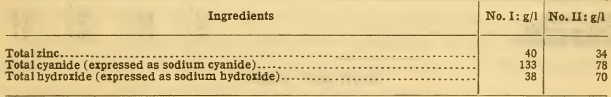To prepare these solutions from zinc cyanide will require:

No. I. 40 x 1.8 = 72 g/l zinc cyanide.
No. II. 34 x 1.8 =61 g/l zinc cyanide.

These are equivalent to:

No. I. 72 x 0.84 = 61 g/l sodium cyanide.
No. II. 61 x 0.84 = 51 g/l sodium cyanide.

Therefore the solutions require:

No. I. 133—61 =72 g/l sodium cyanide.
No. II. 78—51 =27 g/l sodium cyanide.

And the full amounts of sodium hydroxide, viz, 38 g/1 and 70 g/1, respectively.

To prepare them by the use of zinc oxide would require:

No. I. 40 x 1.24 = 50 g/l zinc oxide.
No. II. 34 x 1.24 = 42 g/l zinc oxide.

In No. I, however, only 38 g/l of sodium hydroxide is present; therefore only 38 g/l of zinc oxide may be used. This is equivalent to 38 x 0.8 =30 g/l zinc, leaving 10 g/l of zinc to be added as zinc cyanide; that is, 10 x 1.8 = 18 g/l of zinc cyanide, which is equivalent to 18 x 0.84 = 15 g/l of sodium cyanide; therefore 133 —15 = 118 g/l of sodium cyanide is required; while in No. II, where all the zinc is to be added as zinc oxide, the full amount of sodium cyanide, 78 g/l, is required. In No. I no sodium hydroxide will be required (since it is all supplied by the solution of the zinc oxide); while in No. II, 70 — 42 = 28 g/l of sodium hydroxide is required. The formulas for the preparation of the solutions will therefore be as follows: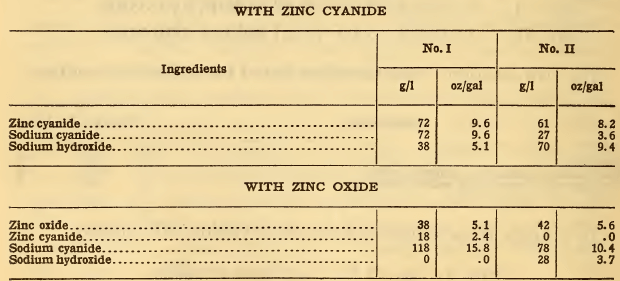The calculation of assumed excess cyanide may be made as follows:

No. I. The total cyanide is equivalent to 133 g/l x 0.33 = 44 g/l zinc. Since this is greater than the zinc content (40 g/l) there must be an excess of cyanide. 40 g/l x 3 = 120 g/l sodium cyanide, therefore there is 133 — 120 = 13 g/l excess sodium cyanide; and the entire amount, that is, 38 g/l, excess sodium hydroxide.

No. II. The total cyanide is equivalent to 78 g/l x 0.33 =26 g/l of zinc. Therefore 34 — 26=8 g/l of zinc is combined with sodium hydroxide. 8 x 2.45=20 g/l of sodium hydroxide is required to combine with 8 g/l of zinc. The solution therefore contains 70—20 = 50 g/l excess sodium hydroxide.
The solutions may therefore be said to contain: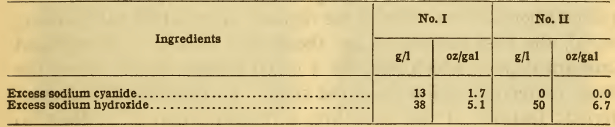### OPERATION OF SOLUTIONS

Since, as shown previously, it is possible to replace zinc cyanide in the customary plating formulas by zinc oxide in amounts up to the desired content of sodium hydroxide, and since in preliminary experiments it was found that a rather high content of sodium hydroxide was desirable, all the solutions employed in these experiments were prepared by the solution of zinc oxide in sodium cyanide, with in some cases the actual addition of sodium hydroxide. It should be clearly understood, however, that any of these solutions may be prepared by the use of zinc cyanide instead of zinc oxide, with appropriate changes in the amounts of the other constituents. The composition recommended will therefore be expressed in terms of the two methods of preparation.

Preliminary experiments upon the solubility of zinc oxide in sodium cyanide showed that although according to the simple equations 81 parts of zinc oxide should dissolve in 98 parts of sodium cyanide—that is in 1.21 times its own weight—considerably more than this proportion of sodium cyanide is actually required to produce a clear solution. This observation is in accordance with the well-known fact that sodium zincate solutions require a considerable excess of alkali, and sodium zinc cyanide solutions an appreciable excess of cyanide to overcome the effects of hydrolysis and dissociation, and to maintain clear solutions. For practical purposes it was found that to produce complete solution the sodium cyanide must be about 1.67 times the weight of the zinc oxide. Additional cyanide and hydroxide were added in some cases.

The effects of composition, concentration, current density, and temperature were determined in a large number of experiments, usually conducted in baths containing about 1200 cc and operated in series with a copper voltameter. Observations were also made upon the conductivity of the solutions, the polarization occurring under the conditions employed, the anode and cathode efficiency, and the appearance and microstructure of the deposits. In the selection of favorable operating conditions, emphasis has been placed upon the structure of the deposits since, other things being equal, the best conditions are those that lead to a fine-grained uniform deposit which will, for a given weight of zinc, exert the most protective action upon the steel. To illustrate the characteristic features of the structure, a magnification of at least 20 diameters is necessary. Even with a pocket lens, however, it is easily possible to distinguish between deposits with fine and coarse crystals, and between those having a dense structure and those having a loose or porous structure. A dark or powdery appearance to the naked eye is evidence of a poor deposit. On the other hand, the “whitest’’ deposits are not necessarily the most dense. Usually the fine dense deposits have a light silvery luster.

The following conclusions may be drawn from the experiments thus far conducted at this Bureau:

## Composition and Concentration

As previously stated, it was found necessary to have 1.67 times as much sodium cyanide as zinc oxide; for example, for 45 g/l (6 oz./gal.) of zinc oxide, 75 g/l (10 oz./gal.) of sodium cyanide are required. Any excess of cyanide above this amount—for example, an excess of 15 g/l (2 oz./gal.)—in every case produced coarse deposits (Fig. 2). Fine¬grained deposits (Fig. 1) can be secured from solutions containing only zinc oxide and sodium cyanide in the above proportions, but to do so requires a relatively high voltage, especially with current densities higher than 1 amp/dm² (9.3 amp/sq. ft.). This was found to be due not so much to a low conductivity of such solutions as to a high polarization, arising from accumulation of insoluble products upon the anodes. This condition was immediately remedied by the addition of small amounts of sodium hydroxide, up to 15 g/l (2 oz./gal.). From such solutions good deposits (Fig. 3) were obtained over a wide range of conditions.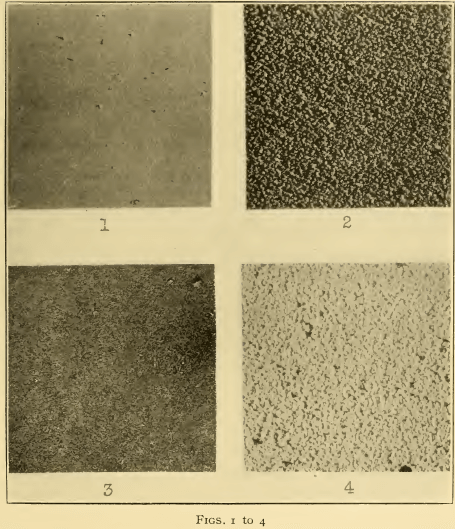In general, with the above proportion of the constituents there is not much effect due to concentration. In dilute solutions at low current densities, or for very thin deposits (as, for example, in a cyanide “strike” solution) the deposits appear to be somewhat finer grained than in the more concentrated solutions. On the other hand, in more concentrated solutions the resistance and polarization are lower, and higher current densities can be employed (for example, up to 3 amp/dm² or 28 amp/sq. ft.). The use of fairly concentrated solutions, containing at least 45 g/l (6 oz./gal.) of zinc oxide, or 36 g/l (4.8 oz./gal.) of metallic zinc, is therefore recommended. There is a decided disadvantage in the presence of carbonates, which should not therefore be introduced into the solution, either by the addition of zinc carbonate or sodium carbonate, or by the use of sodium cyanide or hydroxide containing much carbonate. In general, carbonates tend to produce coarse or striated deposits (Fig. 4). Since some carbonate always forms in such solutions, owing to absorption of carbon dioxide from the air, and to decomposition of the cyanide, it is desirable to remove it periodically, for example, every three or six months at the time of the regular “clean-up.” This can be readily accomplished by the addition of barium cyanide solution, or equally well of a solution containing sodium cyanide and barium hydroxide. The precipitate of barium carbonate which separates should be allowed to settle, after which the clear solution may be siphoned out in the usual way. Carbonates can also be eliminated to a great extent by simply cooling the solution to o° C or 32° F, or to as low a temperature as possible, when sodium carbonate crystallizes out and can be readily removed. While it is not possible to state just what is the maximum permissible content of sodium carbonate, or just how fast it will accumulate in the solution, it is desirable, if possible, to maintain it below 15 g/l (2 oz./gal.).

## Current Density

In general, an increase in the current density up to the point at which the current efficiency falls off, and marked “gassing” occurs, produces a somewhat finer structure. The effects of current densities from 1 to 3 amp/dm² (9 to 28 amp/sq. ft.) are not, however, sufficiently marked to warrant the use of the higher current density except from the obvious advantage of reducing the time required. For average purposes a current density of about 2 amp/dm² (19 amp/sq. ft.) will be satisfactory; on plain objects higher current densities may be employed and for complicated shapes a lower current density must be used to prevent excessive deposition on edges, etc.

## Temperature

In general, it is found that warm solutions produce somewhat coarser deposits than cold solutions. On the other hand, they permit good deposits to be secured at somewhat higher current densities, partly because any gas liberated on the cathode is less likely to adhere at the higher temperatures. The better conductivity of the warm solution permits the use of a lower voltage than in cold solutions to secure any desired current density. For most purposes where there is no necessity for using current densities above 2 amp/dm² (19 amp/sq. ft.) there is, however, little if any advantage in heating the solutions. In any case the temperature should not be allowed to rise above 50° C (122° F); otherwise excessive decomposition of cyanide occurs.

## Conditions Recommended

In suggesting the following conditions of operation it is not implied that these are necessarily the best for practical operation for any given class of work. It is believed, however, that these conditions will serve as a guide in the preparation, operation, and control of such solution. To avoid confusion a definite formula is given, although as previously stated the composition may vary somewhat without producing noticeable effects. For purposes of comparison the concentrations are expressed in terms of the normality, but for convenience of application the values have been rounded off to be equivalent to a simple number of ounces per gallon, making allowances for amounts of impurities likely to be present in the commercial chemicals.

#### 1 Composition.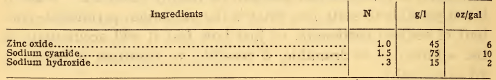The same solution can be prepared from: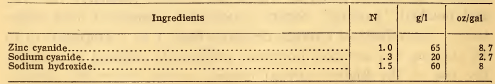Temperature: 20 to 40° C (68 to 104° F.).

#### Current density:

Average about 2 amp/dm² (19 amp/sq. ft.). In using zinc oxide it is desirable to obtain material nearly free from lead, any large amount of which is objectionable in the solutions. In case lead is present it will produce a gray sponge upon the anodes on standing overnight. In such a case a large number of zinc anodes should be suspended in the solution (and cleaned at intervals) until no more lead appears upon them. By this means all the lead can be removed.

Technologic Papers of The Bureau of Standards